# Test: Circles- 2

## 25 Questions MCQ Test Mathematics (Maths) Class 9 | Test: Circles- 2

Description
This mock test of Test: Circles- 2 for Class 9 helps you for every Class 9 entrance exam. This contains 25 Multiple Choice Questions for Class 9 Test: Circles- 2 (mcq) to study with solutions a complete question bank. The solved questions answers in this Test: Circles- 2 quiz give you a good mix of easy questions and tough questions. Class 9 students definitely take this Test: Circles- 2 exercise for a better result in the exam. You can find other Test: Circles- 2 extra questions, long questions & short questions for Class 9 on EduRev as well by searching above.
QUESTION: 1

Solution:
QUESTION: 2

### In the given figure, AC is a diameter of the given circle and ∠BCD = 75o. Then, ∠EAF−∠ABC is equal to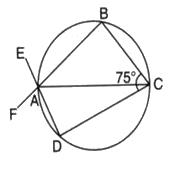Solution: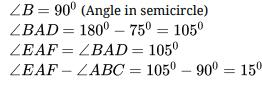QUESTION: 3

### The region between chord and either of the arc is called

Solution:
QUESTION: 4

P is a point on the diameter AB of a circle and CD is a chord perpendicular to AB. If AP = 4 cm and PB = 16 cm, the length of chord CD is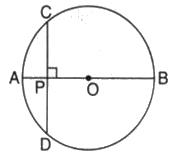Solution:
QUESTION: 5

Arc ABC subtends an angle of 130o at the centre O of the circle. AB is extended to P. Then ∠CBP equals :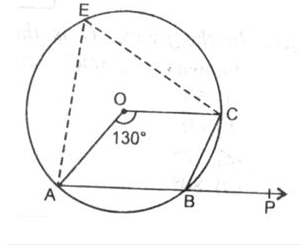Solution:
QUESTION: 6

Chords AB and CD intersect at right angles. If ∠BAC = 40o, then ∠ABD is equal to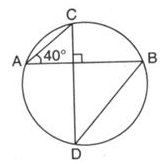Solution:
QUESTION: 7

In the given figure, O is the centre of the circle. ∠OAB and ∠OCB are 40o and 30o respectively. Then, the measure of ∠AOC is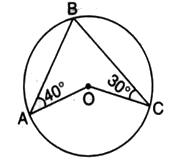Solution: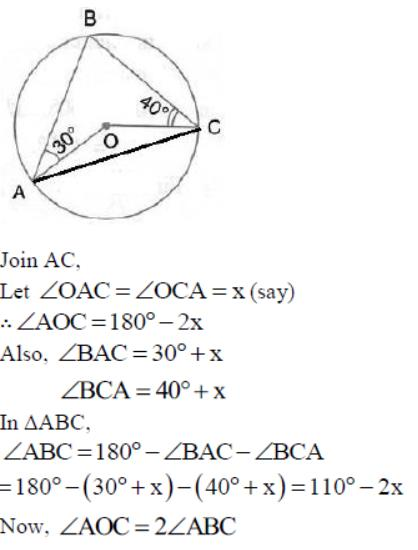(Angle at the centre is double the angle at the circumference subtended by the same chord)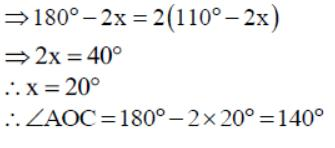QUESTION: 8

In the given, AB is side of regular five sided polygon and AC is a side of a regular six sided polygon inscribed in the circle with centre O. AO and CB intersect at P, then ∠APB is equal to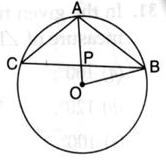Solution:
QUESTION: 9

In the given figure, if ∠AOB = 80o and ∠ABC = 30o , then ∠CAO is equal to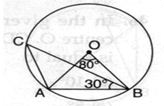Solution:

2ACB=AOB
ACB=40
CAB+ACB+ABC=180
CAB=180-70
CAB=110
OAB=OBA

AOB+OAB+OBA=180
2OBA=100
OBA=50

CAO=CAB-OBA=110-5O
CAO=60o

QUESTION: 10

In the figure, O is the centre of eh circle and ∠AOB = 80o. The value of x is :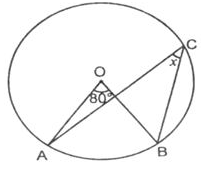Solution:
QUESTION: 11

In the given figure if OA = 5 cm, AB = 8 cm and OD is perpendicular to AB, then CD is equal to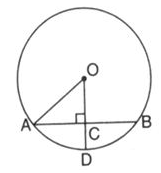Solution:
QUESTION: 12

If ∠OAB = 40o, then the measure of ∠ACB is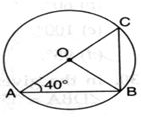Solution:
QUESTION: 13

Chords AD and BC intersect each other at right angles at point P. ∠DAB = 35o, then ∠ADC is equal to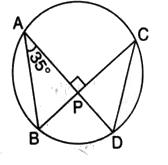Solution:
QUESTION: 14

In the given figure, AB is a diameter of the circle APBR. APQ and RBQ are straight lines. If ∠A = 35o and , then the measure of ∠PBR is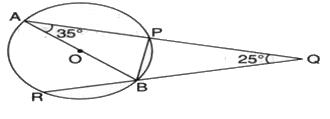Solution:
QUESTION: 15

In the figure, O is the center of the circle. If ∠OAB = 40o, then ∠ACB is equal to :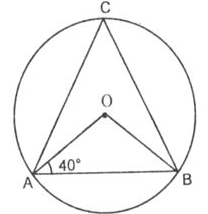Solution:
QUESTION: 16

X is a point on a circle with centre O. If X is equidistant from the two radii OP, OQ, then arc PX : arc PQ is equal to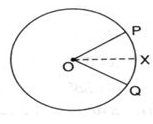Solution: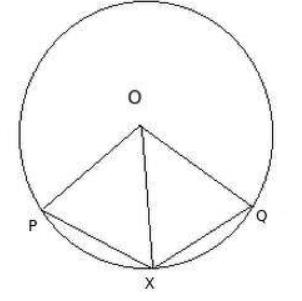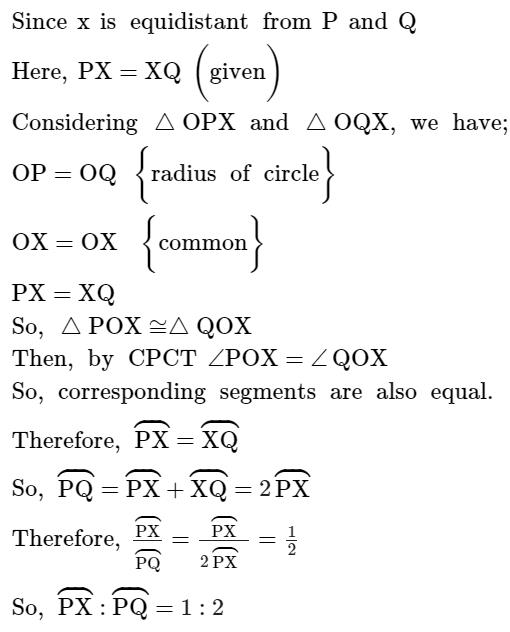QUESTION: 17

BC is a diameter of the circle and ∠BAO = 60o . Then ∠ADC is equal to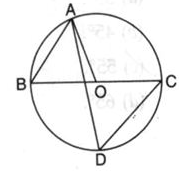Solution:
QUESTION: 18

What fraction of the whole circle is minor arc RP in the given figure ?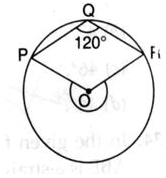Solution:
QUESTION: 19

In the given figure, AD is the diameter of the circle and AE = DE. If ∠ABC = 115o, then the measure of ∠CAE is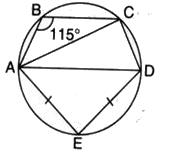Solution:
QUESTION: 20

In the figure, if ∠DAB = 60o, ∠ABD = 50o, then ∠ACB is equal to :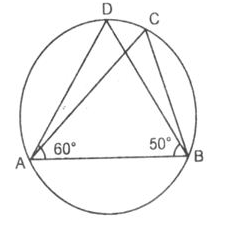Solution:
QUESTION: 21

If ABCD is a cyclic trapezium in which AD ║ BC and ∠B = 60o, then ∠BCD is equal to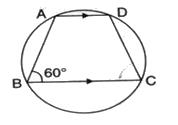Solution:
QUESTION: 22

In the given figure, O is the centre of the circle and ∠AOC = 130o. Then ∠ABC is equal to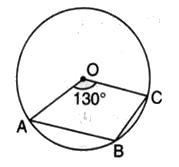Solution:

360o - 130o =230o

Therefore, angle ABC =1/2reflex angle AOC

Angle ABC =1/2 230o

So, angle ABC =115o

QUESTION: 23

In the given circle, O is the centre and ∠BDC = 42o. Then, ∠ACB is equal to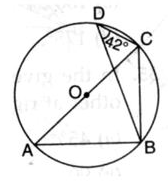Solution:

In ∆ BDC and ∆ BAC
Angle BAC = BDC
(angle made on same segment BC)
Since ABC is making right angle (90)
So,
In ∆ABC
ABC +BAC+ACB=180
(angle sum property of triangle)
90+42+ACB=180
ACB=180-132
ACB=48o

QUESTION: 24

AOB is the diameter of the circle. If ∠AOE = 150o, then the measure of ∠CBE is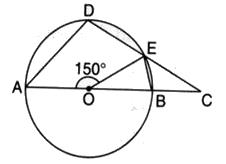Solution:
QUESTION: 25

In the given figure, a circle is centred at O. The value of x is :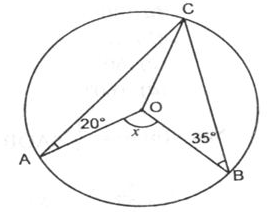Solution: